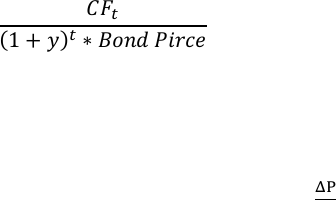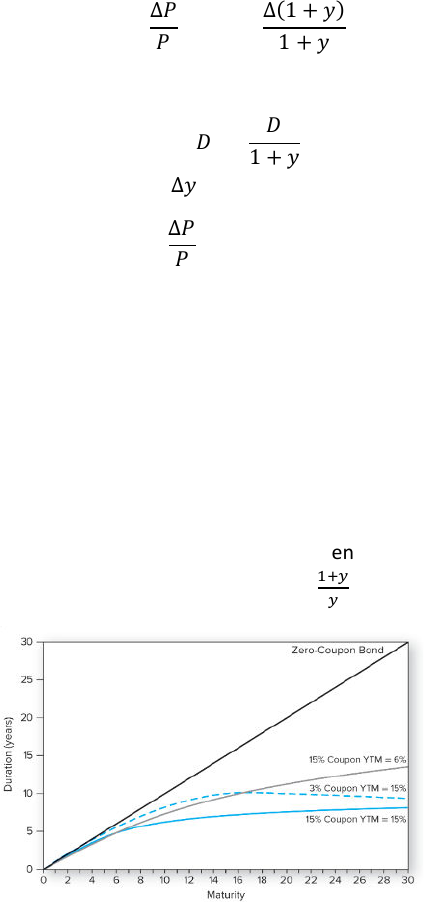Class Notes (1,100,000)
CA (650,000)
WLU (20,000)
BU (4,000)
BU473 (10)
Lecture 16

# BU473 Lecture Notes - Lecture 16: Interest Rate Risk, Discounted Cash Flow, Cash Flow

Department
Course Code
BU473
Professor
David Cimon
Lecture
16

This preview shows page 1. to view the full 5 pages of the document.BU-473 Lecture 16
Interest Rate Sensitivity
We can summarize changes in bond prices with respect to changes in interest rates in a few
statements, known as Malkiel’s Bond-Pricing Theorems:
1. Bond prices and yields are inversely related; as yields increase, bond prices fall; as yields fall,
bond prices increase.
2. An increase in a bond’s yield to maturity results in a smaller price change than a decrease in
yield of equal magnitude.
3. Prices of long-term bonds tend to be more sensitive to interest rate changes than prices of short
term bonds.
4. The sensitivity of bond prices to changes in yields increases at a decreasing rate as maturity
increases. In other words, interest rate risk is less than proportional to bond maturity.
5. The interest rate risk is inversely relation to the bond’s coupon rate. Prices of low coupon bonds
are more sensitive to changes in interest rates than prices of high-coupon bonds.
Duration
Bond maturity and cash flow timing pay key roles in price changes due to interest rates
Ideally, we’d have a method to measure this using a single stat that summarized the relative
maturity of all of a bond’s cash flows, rather than just its face value
To do this, we’ll measure the average timing of all bond cash flow, Duration
Macaulay Duration
Most common form of duration measurement
Macaulay’s duration weights the time-to-maturity of a bond by the relative, discounted cash
flow in each time period
Macaulay’s Duration:
 

Where t is the timing of each cash flow in years.
The weight for each year t is given by:
 
Modified Duration
Another important concept is the relative change in a bond’s price in response to a change in
yields
We can show that for a yield change of , the percentage change in price
can be linearly
approximated as:

Unlock to view full version

Only page 1 are available for preview. Some parts have been intentionally blurred. 
To make this calculation easier, we can modify our duration measure to a second measure as
modified duration:
Replacing D with D* and  with , we get:
 
Duration in Practice
There are a few rules for how a bond’s characteristics affect its duration
1. The duration of a zero-coupon bond equals its time to maturity.
2. Holding maturity constant, a bond’s duration is lower when its coupon is higher.
3. Holding coupon rate constant, a bond’s duration generally increases with time to maturity.
Duration always increases with maturity if the bond is trading at or above par.
a. NOTE: A bond selling at a very high discount may have a duration that decreases with
maturity.
4. All else equal, the duration of a coupon bond is higher when the bond’s YTM is lower.
5. The duration of a perpetuity with a yield of y is given by
.
Changes in Duration: Convexity
An issue with the duration formula is that it’s a linear approximation of the change in bond price
as a result of a yield change
When yields change, the bond’s price responds non-linearly to this change
Thus, duration doesn’t fully represent the potential change in a bond’s price
To improve the approximation of a bond’s price change we need to use another measure that
we refer to as convexity
Convexity
We measure the change in the slope of the pricing curve using a measure called convexity.
Convexity can be calculated as: